C Program to Convert Kilogram to Gram

In this tutorial you will learn about the C Program to Convert Kilogram to Gram and its application with practical example.

C Program to Convert Kilogram to Gram

In this tutorial, we will learn to create a C program that will Convert Kilogram to Gram in C programming.

Prerequisites

Before starting with this tutorial we assume that you are best aware of the following C programming topics:

• Operators in C Programming.
• Basic Input and Output function in C Programming.
• Basic C programming.

Program to Convert Kilogram to Gram

As we all know the c is a very powerful language. With the help of c programming language, we can make many programs. We cal perform many input-output operations using c programming. In today’s tutorial, we take the input in kilograms from the user and convert it into grams. With the help of c programming, we can perform many conversion operations.

With the help of this program, we can Convert Kilogram to Gram.

Output:-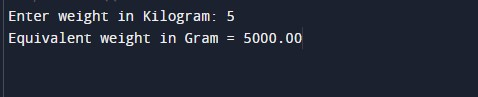In the above program, we have first initialized the required variable.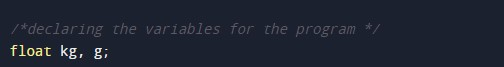• kg = it will hold the float value for the program as an input from the user.
• g = it will hold the float value that will hold the grams converted in the program.

Taking the input from the user in the form of kilograms.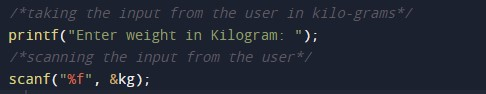Converting the kilograms to grams.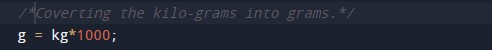Printing the converted output from kilograms to grams.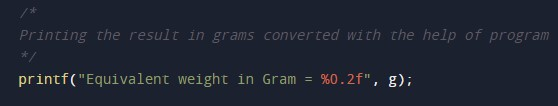In this tutorial we have learn about the C Program to Convert Kilogram to Gram and its application with practical example. I hope you will like this tutorial.